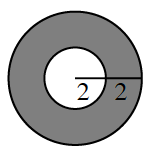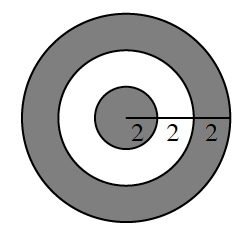### Home > CCA2 > Chapter 2 > Lesson 2.1.4 > Problem2-56

2-56.

A dart hits each of these dartboards at random. What is the probability that the dart will land in the unshaded area?

1.What is the area of the large circle? What about the small circle?

After you find these areas, create a ratio that will give you the probability of hitting the shaded region.

$P(\text{land in unshaded area})=\frac{1}{4}$

1.Find the area of the unshaded region. What is the radius of the circle that is the unshaded region and the small, inner circle?

Subtract the area of the small, inner circle.

Find the area of the large circle and create a ratio, like you did in part (a).